$$\def\loading{......LOADING......Please Wait} \def\RR{\bf R} \def\real{\mathbb{R}} \def\bold#1{\bf #1} \def\d{\mbox{Cord}} \def\hd{\widehat \mbox{Cord}} \DeclareMathOperator{\cov}{cov} \DeclareMathOperator{\var}{var} \DeclareMathOperator{\cor}{cor} \newcommand{\ac}{\left\{#1\right\}} \DeclareMathOperator{\Ex}{\mathbb{E}} \DeclareMathOperator{\diag}{diag} \newcommand{\bm}{\boldsymbol{#1}} \def\wait{......LOADING......Please Wait}$$

## Granger Mediation Analysis for Multiple Time Series

### Xi (Rossi) LUO

The University of Texas
Health Science Center
School of Public Health
Dept of Biostatistics
and Data Science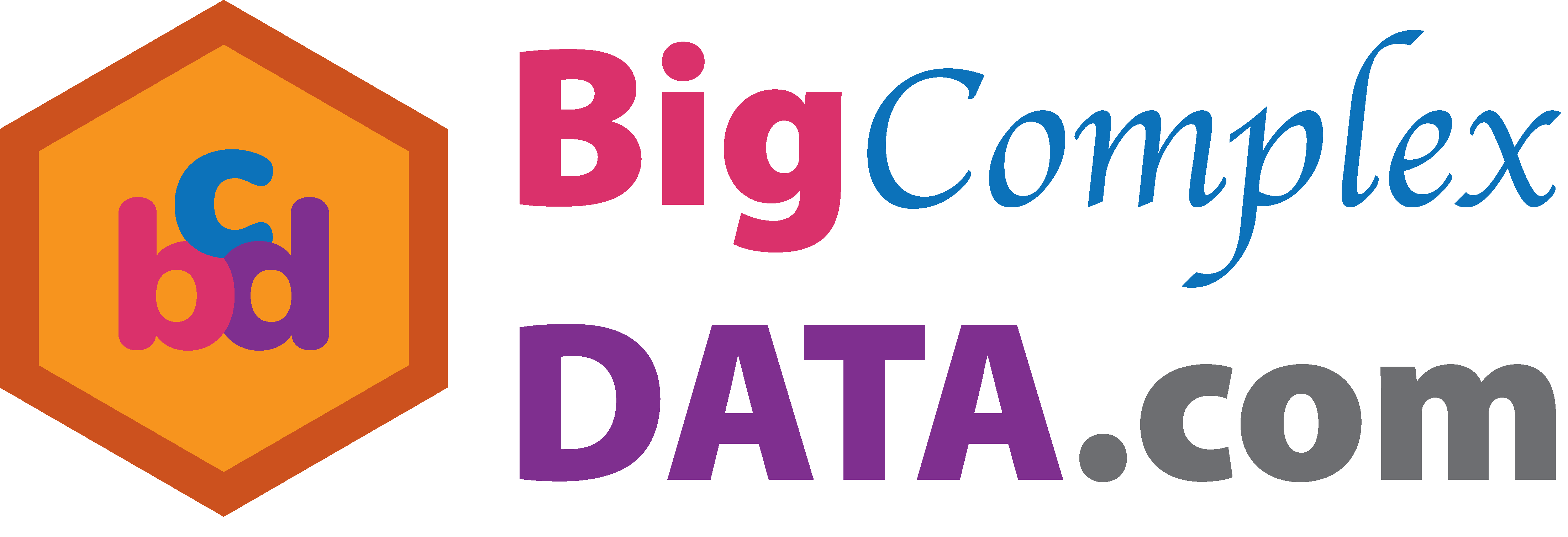August 6, 2020

Funding: NIH R01EB022911, P01AA019072, P20GM103645, P30AI042853; NSF/DMS (BD2K) 1557467

# Slides viewable on web: bit.ly/mediationjsm20 or

## Co-AuthorYi Zhao
Assistant Prof
Indiana Univ

## fMRI Experiments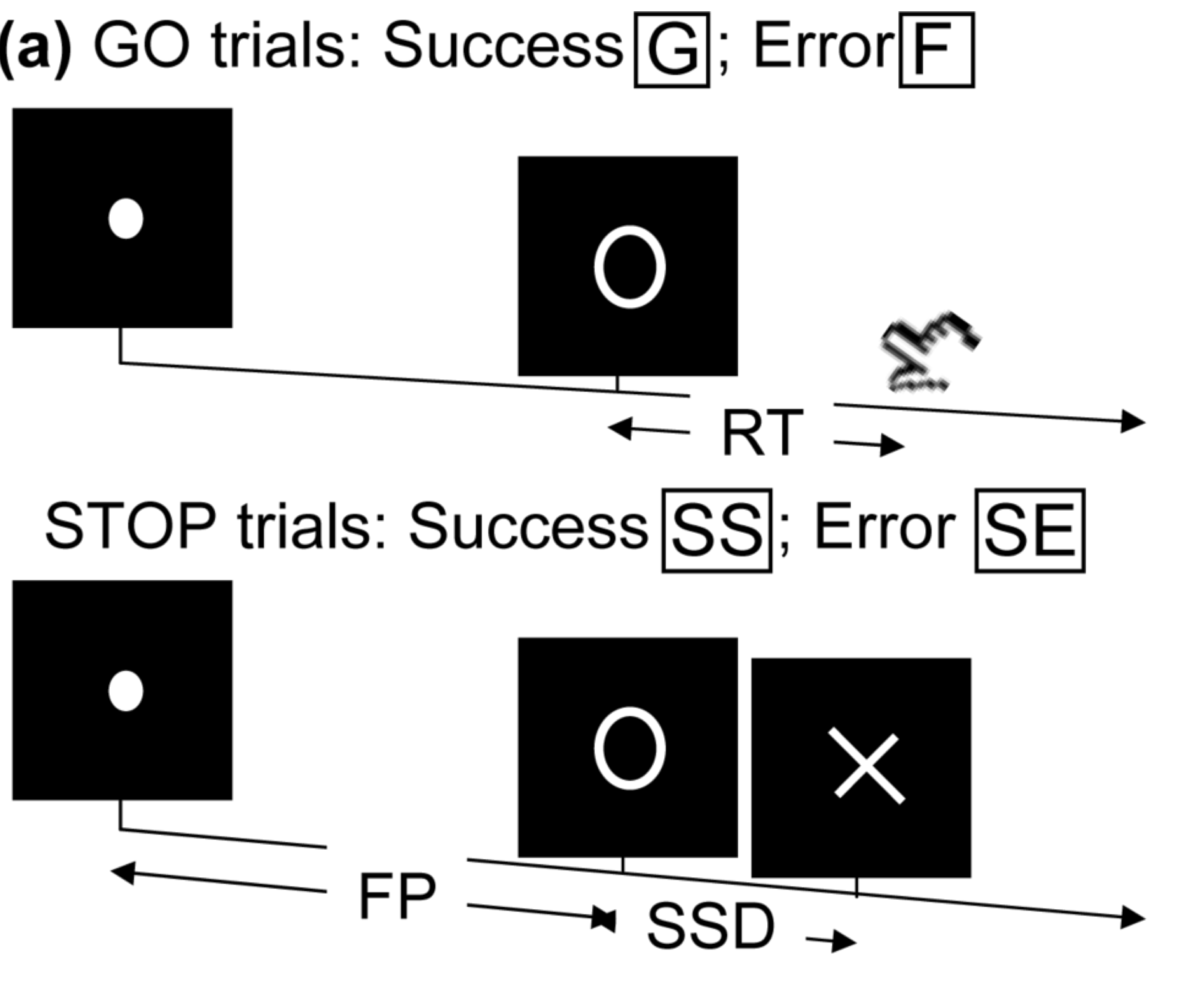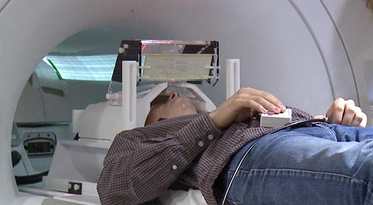• press button if "go";
• withhold pressing if "stop"
• Not resting-state: "do nothing" during scanning

Goal: infer brain activation and connectivity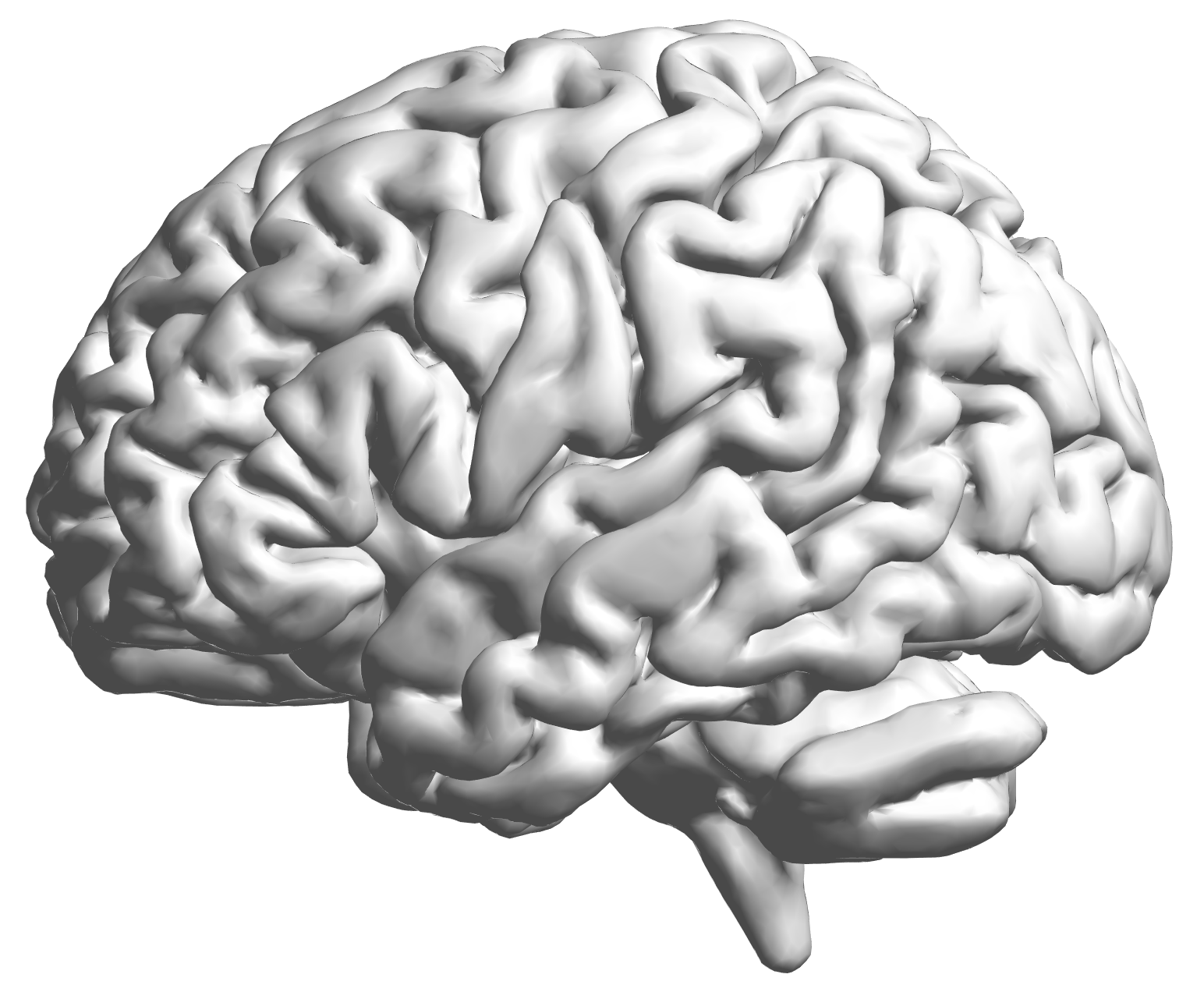fMRI data: blood-oxygen-level dependent (BOLD) signals from each cube/voxel (~millimeters), $10^5$ ~ $10^6$ voxels in total.

## Multilevel fMRI Studies

Sub 1, Sess 1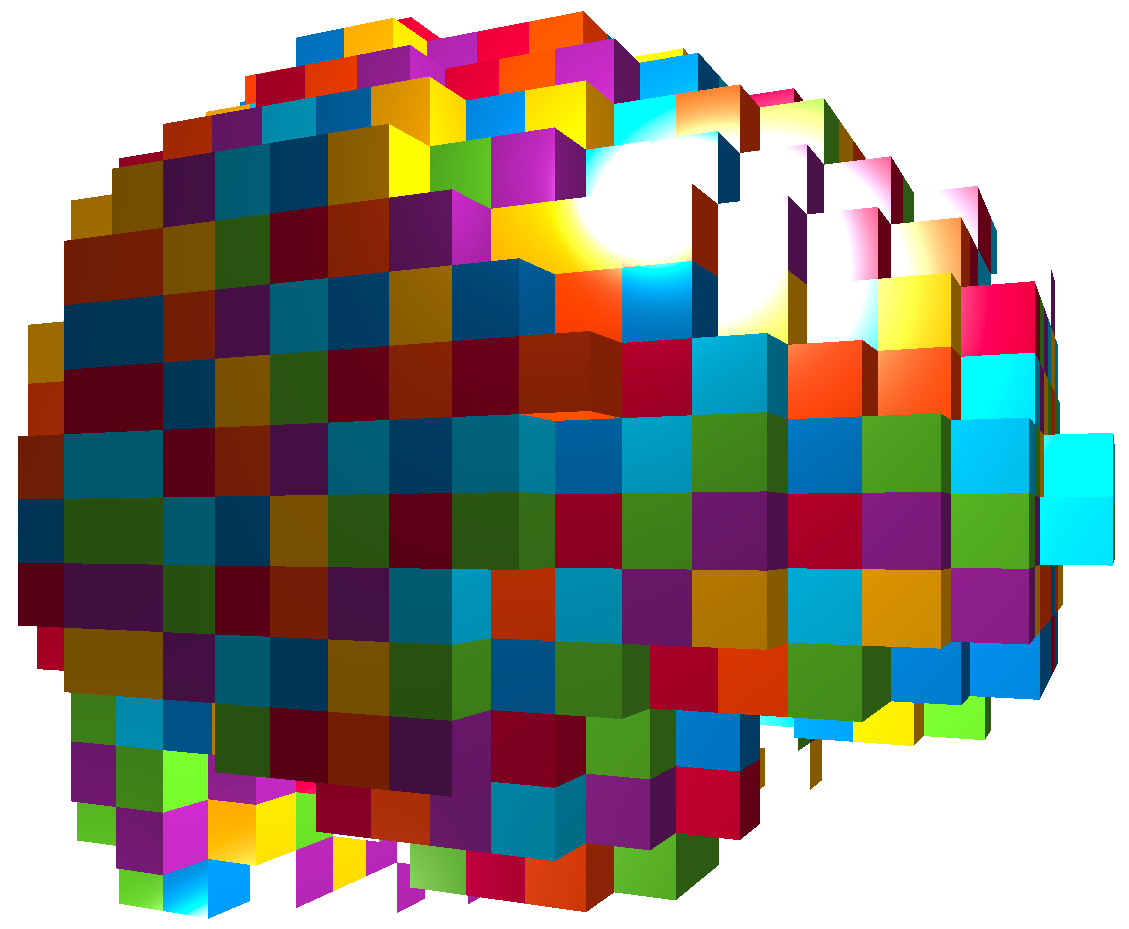Time 12~200

Sub i, Sess jSub ~100, Sess ~4Large, multilevel (subject, session, voxel) data
e.g. $1000 \times 4 \times 300 \times 10^6 \approx 1$ trillion data points

## Raw Data: Motor Region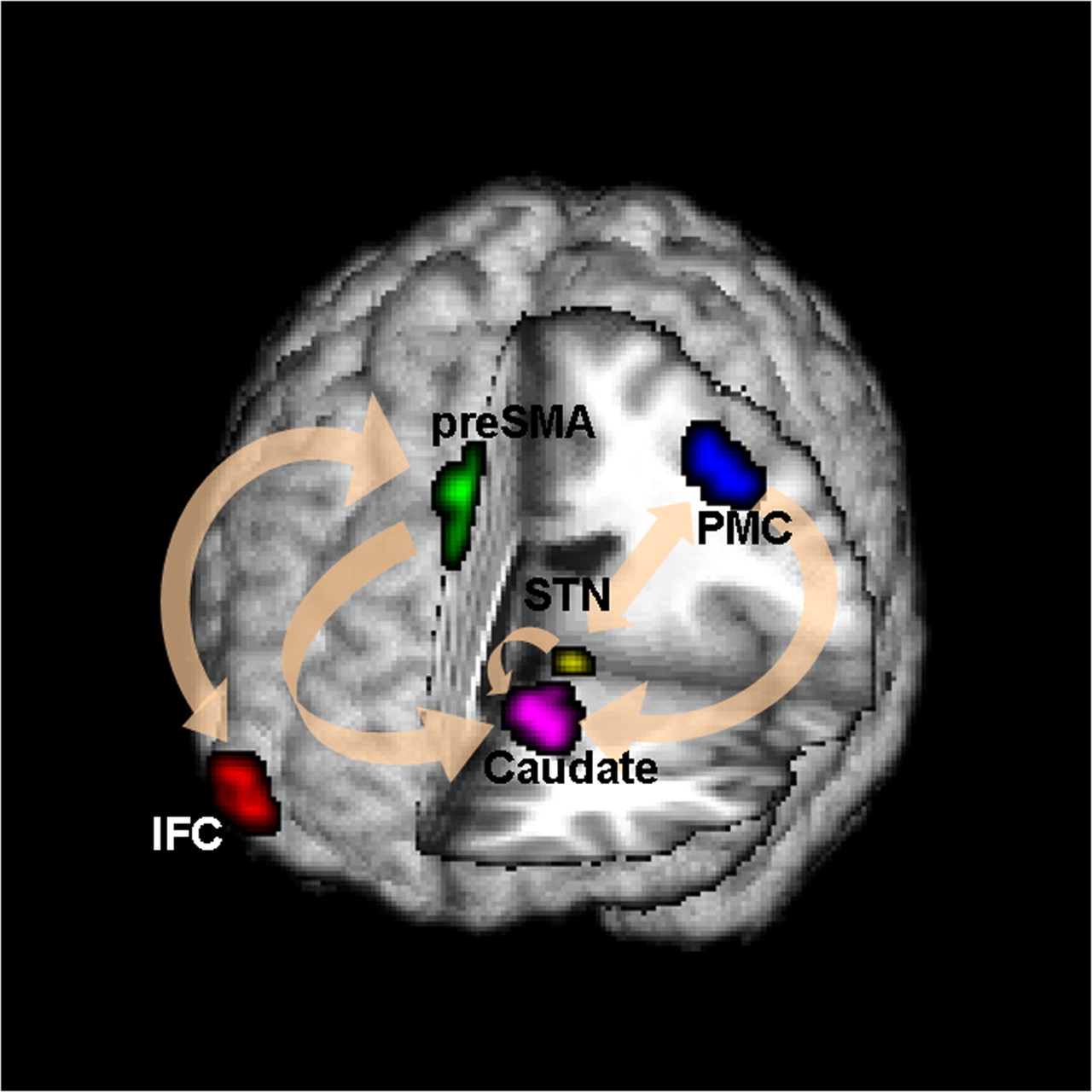Time (seconds)

Black: fMRI BOLD activity
Blue: stop onset times; Red: go onset times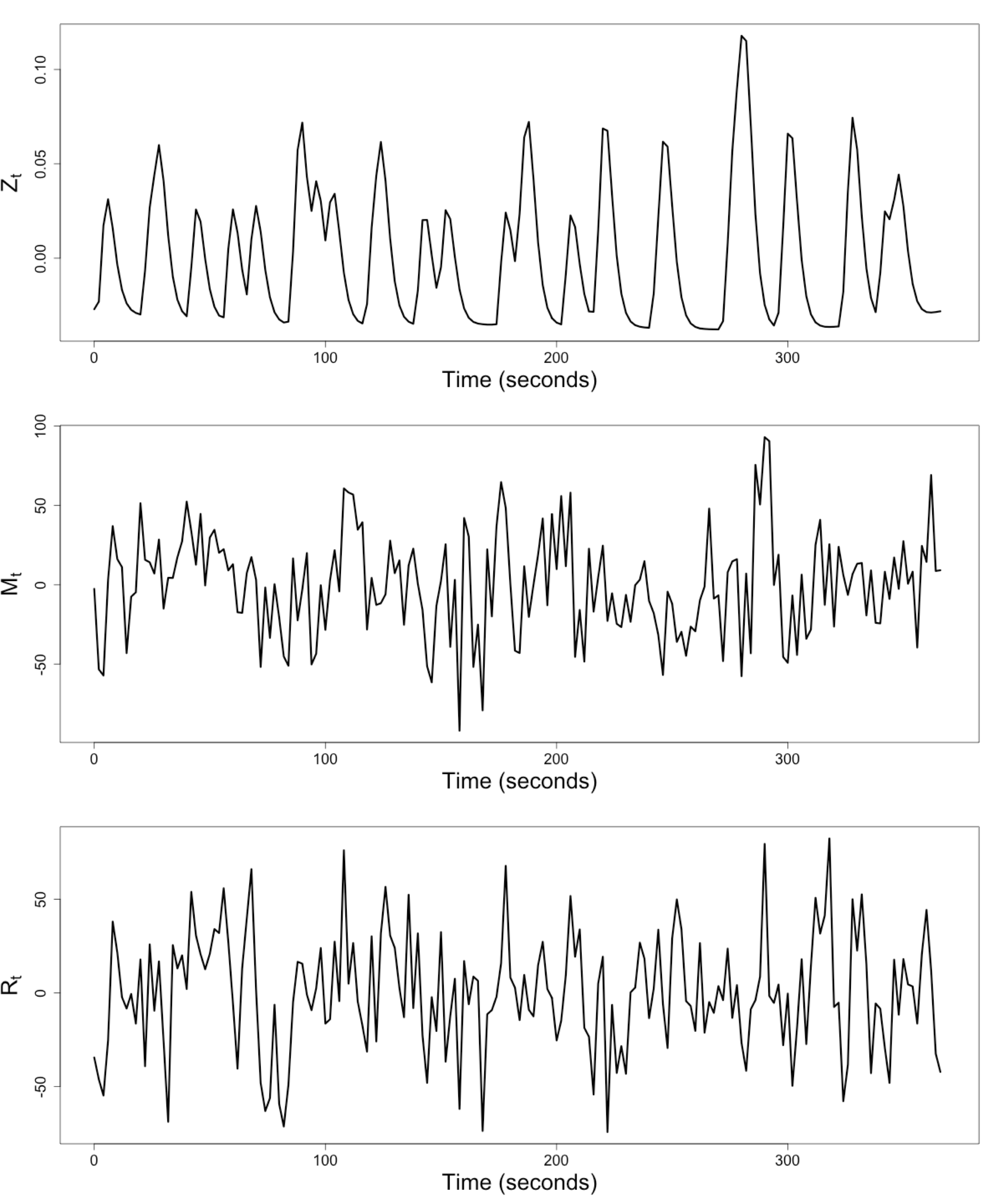$Z_t$: Stimulus onsets convoluted with Canonical HRF

$M_t$, $R_t$: fMRI time series from two brain regions

## Review: Granger Causality/VAR

• Given two (or more) time series $x_t$ and $y_t$ \begin{align*} x_t &= \sum_{j=1}^p \psi_{1j} x_{t-j} + \sum_{j=1}^p \phi_{1j} y_{t-j} + \epsilon_{1t} \\ y_t &= \sum_{j=1}^p \psi_{2j} y_{t-j} + \sum_{j=1}^p \phi_{2j} x_{t-j} + \epsilon_{2t} \end{align*}
• Also called vector autoregressive models
• $y$ Granger causes $x$ if $\phi_{1j} \ne 0$ Granger, 69
• Models pair-wise connections not pathways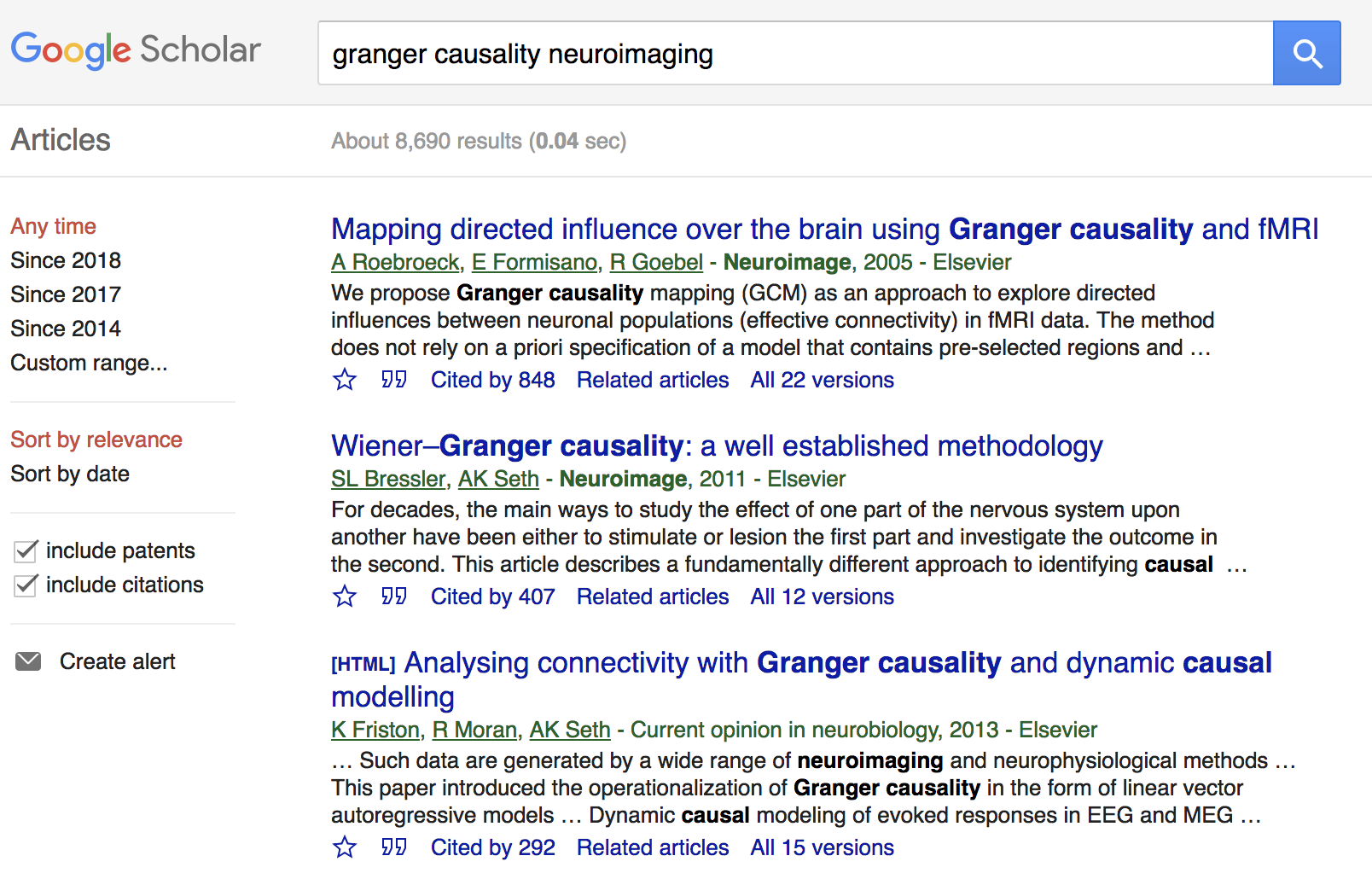## Granger Causality/VAR

• Granger Causality (VAR) popular for fMRI
• Over 10,000 google scholar results on "granger causality neuroimaging", as of May 29, 2019
• Models multiple stationary time series
• AR($p$) (small $p$) fits fMRI well Lingdquist, 08
• Cannot model stimulus effects

## Conceptual Brain Model with StimulusGoal: quantify effects stimulipreSMAPMC regions Duann, Ide, Luo, Li (2009). J of Neurosci

## Model: Mediation Analysis and SEM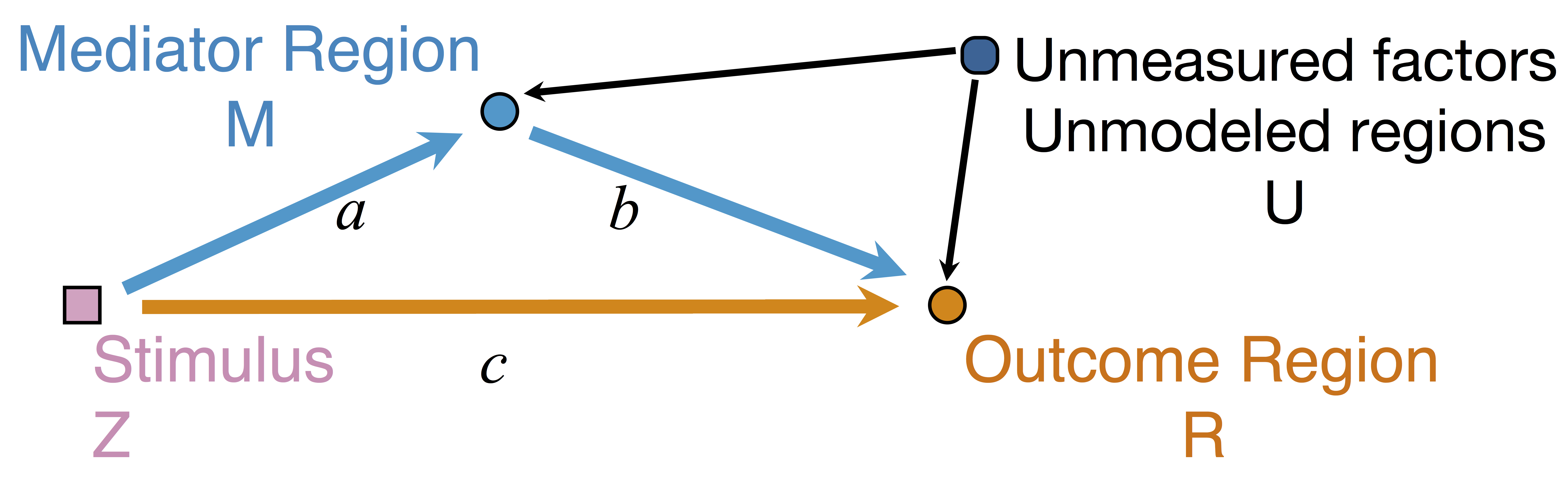\begin{align*}M &= Z a + \overbrace{U + \epsilon_1}^{E_1}\\ R &= Z c + M b + \underbrace{U g + \epsilon_2}_{E_2}, \quad \epsilon_1 \bot \epsilon_2\end{align*}
• Indirect effect: $a \times b$; Direct effect: $c$
• Correlated errors: $\delta = \cor(E_1, E_2) \ne 0$ if $U\ne 0$

## Mediation Analysis in fMRI

• Mediation analysis (usually assuming $U=0$)Baron&anp;Kenny, 86; Sobel, 82; Holland 88; Preacher&Hayes 08; Imai et al, 10; VanderWeele, 15;...
• Parametric Wager et al, 09 and functional Lindquist, 12 mediation, under (approx.) independent errors
• Stimulus $\rightarrow$ brain $\rightarrow$ user reported ratings, one brain mediator
• Assuming $U=0$ between ratings and brain
• Multiple mediator and multiple pathways
• Dimension reduction by arXiv1511.09354Chen, Crainiceanu, Ogburn, Caffo, Wager, Lindquist, 15
• Pathway Lasso penalization Zhao, Luo, 16
• This talk: integrating Granger causality and mediation analysis

# Model & Method

## Our Mediation Model

\begin{align*}M_{t} = Z_{t} a + E_{1t},\quad R_t = Z_t c + M_t b + E_{2t}\end{align*}
• Temporal VAR errors \begin{align*} E_{1t}&=& \sum_{j=1}^{p}\left(\omega_{11_{j}}E_{1,t-j}+\omega_{21_{j}}E_{2,t-j}\right)+\epsilon_{1t} \\ E_{2t}&=&\sum_{j=1}^{p}\left(\omega_{12_{j}}E_{1,t-j}+\omega_{22_{j}}E_{2,t-j}\right)+\epsilon_{2t} \end{align*}
• Spatial errors: $\epsilon_{1t}, \epsilon_{2t}$ $$\begin{pmatrix} \epsilon_{1t} \\ \epsilon_{2t} \end{pmatrix}\sim\mathcal{N}\left(\boldsymbol{\mathrm{0}},\boldsymbol{\Sigma}\right), \quad \boldsymbol{\Sigma}=\begin{pmatrix} \sigma_{1}^{2} & \delta\sigma_{1}\sigma_{2} \\ \delta\sigma_{1}\sigma_{2} & \sigma_{2}^{2} \end{pmatrix}$$

## Equivalent Form

\begin{align*} \scriptsize M_{t}& \scriptsize =Z_{t}A+\sum_{j=1}^{p}\left(\phi_{1j}Z_{t-j}+\psi_{11_{j}}M_{t-j}+\psi_{21_{j}}R_{t-j}\right)+\epsilon_{1t} \\ \scriptsize R_{t}& \scriptsize =Z_{t}C+M_{t}B+\sum_{j=1}^{p}\left(\phi_{2j}Z_{t-j}+\psi_{12_{j}}M_{t-j}+\psi_{22_{j}}R_{t-j}\right)+\epsilon_{2t} \end{align*}
• Nonzero $\phi$'s and $\psi$'s denote the temporal influence from stimulus to mediator/outcome and etc
• $A$, $B$, $C$ are causal following a similar proof in Sobel, Lindquist, 04

## Defining Indirect/Direct Effects

• The (natural) indirect/direct effects for time series can be defined similarly for time series
• $$\mathrm{AIE}(z_{i},z_{i}^{\prime}) =(z_{i}-z_{i}^{\prime})AB, \quad \mathrm{ADE}=(z_{i}-z_{i}^{\prime})C.$$
• We established causality using potential outcomes.
• Ours also model time series and multilevel dependence

## Causal Conditions

• The treatment randomization regime is the same across time and participants.
• Models are correctly specified, and no treatment-mediator interaction.
• At each time point $t$, the observed outcome is one realization of the potential outcome with observed treatment assignment $\mathbf{\bar S}_{t}$, where $\mathbf{ \bar S}_{t}=( \mathbf{S}_{1},\dots,\mathbf{S}_{t})$.
• The treatment assignment is random across time.
• The causal effects are time-invariant.
• The time-invariant covariance matrix is not affected by the treatment assignments.

## Estimation: Conditional Likelihood

• The full likelihood for our model is too complex
• Given the initial $p$ time points, the conditional likelihood is \begin{align*} & \tiny \ell\left(\boldsymbol{\Theta},\delta~|~\mathbf{Z},\mathcal{I}_{p}\right) = \sum_{t=p+1}^{T}\log f\left((M_{t},R_{t})~|~\mathbf{X}_{t}\right) \\ & \tiny = -\frac{T-p}{2}\log\sigma_{1}^{2}\sigma_{2}^{2}(1-\delta^{2})-\frac{1}{2\sigma_{1}^{2}}\|\mathbf{M}-\mathbf{X}\boldsymbol{\theta}_{1}\|_{2}^{2} \\ & \tiny -\frac{1}{2\sigma_{2}^{2}(1-\delta^{2})}\|(\mathbf{R}-\mathbf{M}B-\mathbf{X}\boldsymbol{\theta}_{2})-\kappa(\mathbf{M}-\mathbf{X}\boldsymbol{\theta}_{1})\|_{2}^{2} \end{align*}

## Full Model for Multilevel Data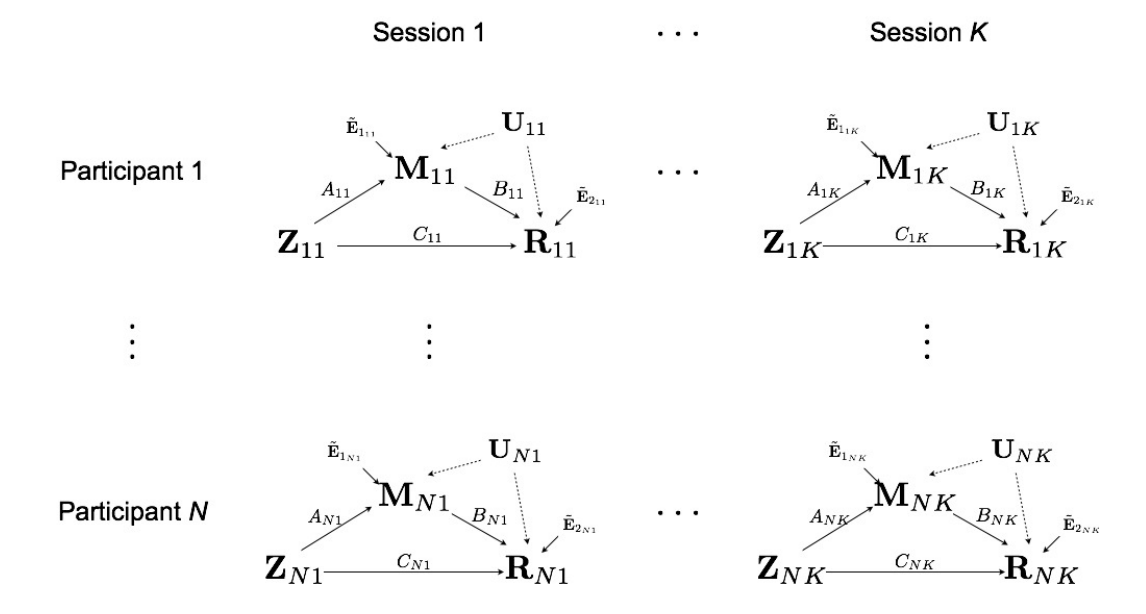## Multilevel Data: Two-level Likelihood

• Second level model, for each subject $i$ $$(A_i,B_i,C_i) = (A,B,C) + (\eta^A_i, \eta^B_i, \eta^C_i)$$ where errors $\eta$ are normally distributed
• The two level likelihood is conditional convex
• Two-stage fitting: plug-in estimates from the first level
• Block coordinate fitting: jointly optimize first level likelihood + second level likelihood
Theorem: Assume assumptions (A1)-(A6) are satisfied. Assume $\mathbb{E}(Z_{i_{t}}^{2})=q\lt \infty$, for $i=1,\dots,N$. Let $T=\min_{i}T_{i}$.
1. If $\boldsymbol{\Lambda}$ is known, then the two-stage estimator $\hat{\delta}$ maximizes the profile likelihood of model asymptotically, and $\hat{\delta}$ is $\sqrt{NT}$-consistent.
2. If $\boldsymbol{\Lambda}$ is unknown, then the profile likelihood of model has a unique maximizer $\hat{\delta}$ asymptotically, and $\hat{\delta}$ is $\sqrt{NT}$-consistent, provided that $1/\varpi=\bar{\kappa}^{2}/\varrho^{2}=\mathcal{O}_{p}(1/\sqrt{NT})$, $\kappa_{i}=\sigma_{i_{2}}/\sigma_{i_{1}}$, $\bar{\kappa}=(1/N)\sum\kappa_{i}$, and $\varrho^{2}=(1/N)\sum(\kappa_{i}-\bar{\kappa})^{2}$.
Using the two-stage estimator $\hat{\delta}$, the CMLE of our model is consistent, as well as the estimator for $\mathbf{b}=(A,B,C)$.

## Theory: Summary

• Under regularity conditions, $N$ subs, $T$ time points
• Our $\hat \delta$ is $\sqrt{NT}$-consistent
• This relaxes the unmeasured confounding assumption in mediation analysis
• Our $(\hat{A},\hat{B}, \hat{C})$ is also consistent

# Simulations & Real Data

## Comparison

• Our methods: GMA-h and GMA-ts
• Previous methods: BK Baron & Kenny, MACC Zhao and Luo, KKB Kenny et al
• Other methods do not model the temporal correlations or time series like ours

## SimulationsLow bias for $AB$Low bias for temporal cor

Gray dash lines are the truth

GMA performs the best, and recovers the temporal correlations

## Real Data Experiment

• Public data: OpenFMRI ds30
• Stop-go experiment: withhold (STOP) from pressing buttons
• Expect "STOP" stimuli to deactivate brain region M1
• Goal: quantify the role of region preSMA

## Result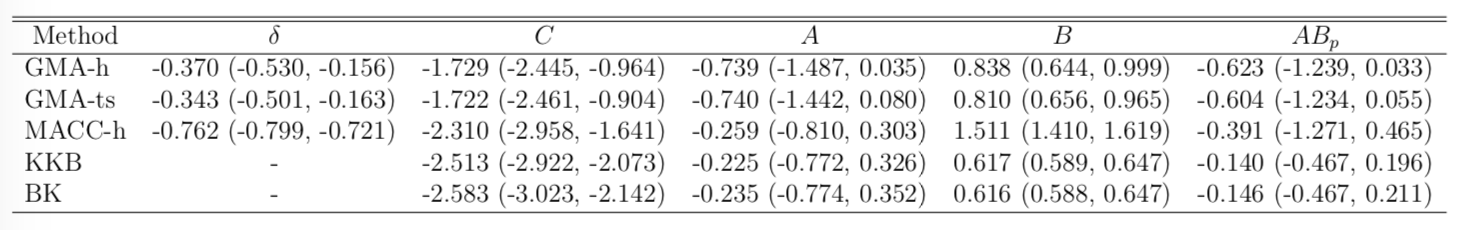• STOP deactivates M1 directly ($C$) and indirectly ($AB$)
• preSMA mediates a good portion of the total effect
• Help resolve the debates among neuroscientists
• Other methods under-estiamte the effects
• Novel feedback findings: M1 → preSMA after lag 1 and 2 (not shown)

## Discussion

• Mediation analysis for multiple time series
• Method: Granger causality + mediation
• Optimizing complex likelihood
• Theory: identifiability, consistency
• Causal assumptions based on potential outcomes
• Result: low bias and improved accuracy
• Extension: functional mediation
• Paper in Biometrics 2019 at 10.1111/biom.13056
• CRAN pkg: gma and references within